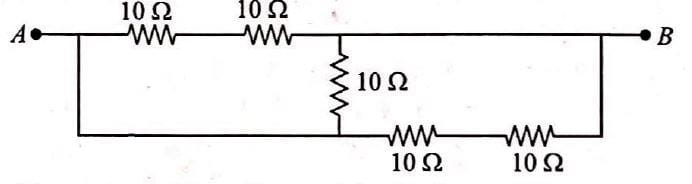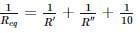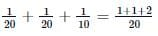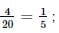Five equal resistances of 10Ω are connected between A and B as shown in figure. The resultant resistance is• a)
10Ω
• b)
• c)
15Ω
• d)

### Related TestCiel Knowledge Feb 08, 2022According to the given circuit 10Ω and 10Ω resistances are connected in series.
∴ R′ = 10 + 10 = 20Ω
Again 10Ω and 10Ω resistances are connected in series
∴ R′′ = 10 + 10 = 20Ω
R′, R′′ and 10Ω all connected in parallel than
∴Req = 5Ω

According to the given circuit 10Ω and 10Ω resistances are connected in series.∴ R′ = 10 + 10 = 20ΩAgain 10Ω and 10Ω resistances are connected in series∴ R′′ = 10 + 10 = 20ΩR′, R′′ and 10Ω all connected in parallel than∴=Req= 5Ω
Do you know? How Toppers prepare for NEET Exam
With help of the best NEET teachers & toppers, We have prepared a guide for student who are preparing for NEET : 15 Steps to clear NEET Exam